It’s World Statistics Day! To honor the theme of the day, the JMP User Community is having conversations about the importance of trust in statistics and data. And we want to hear from you! Tell us the steps you take to ensure that your data is trustworthy.
Choose Language Hide Translation Bar

## Transforming distributions to highlight spread of values

Dear JMP Community,

Machine info: W7 Enterprise 64-bit, JMP Pro 14.1.0

I am interested in transforming a column of data to help highlight the distribution in values within binning ranges. The original data are integers and more or less normally distributed (see Y3 distribution below), but I'd like to transform the data in such a way that it takes the data in a bin range and spreads it out so as to better capture the range of data within a bin (see Y2 distribution). I am including several images to help explain what I'm trying to do -- they are artificial images showing the link, just there to show the desired outcome.

I don't think it's something quite as easy as doing a "center" or "standardize" transformation as neither of those really spread out the data enough (see the highlighted portions in the images). Nor do the Box Cox, or the log transforms. Maybe it's something that would require more than one transformation?

Any suggestions are much appreciated.

Thanks!,

DS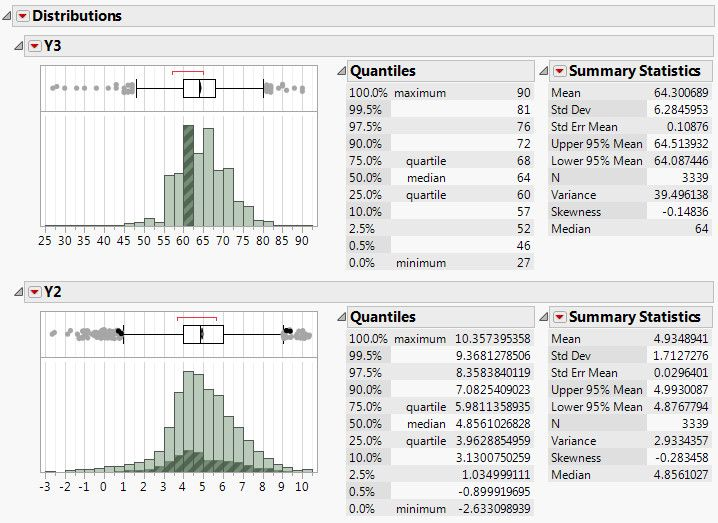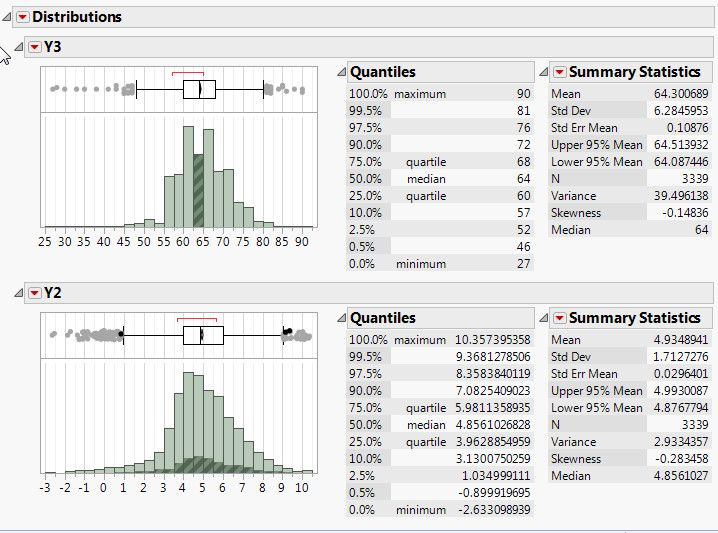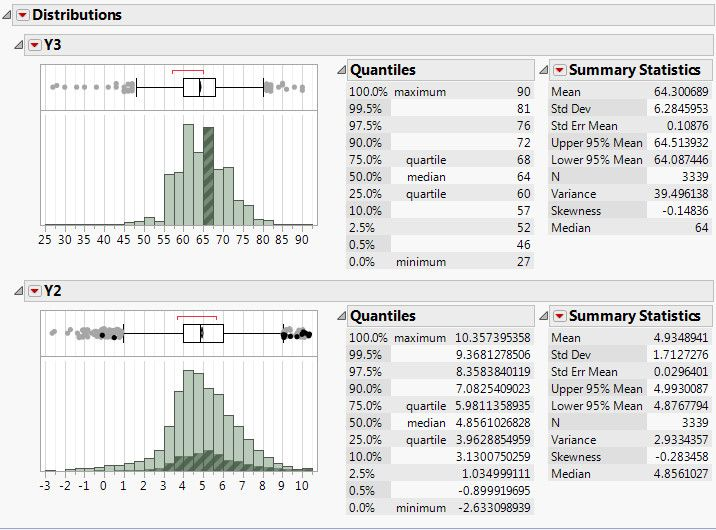5 REPLIES 5
Highlighted

## Re: Transforming distributions to highlight spread of values

If you want to look at a specific range of the data in a histogram, and to change it's binning precision level, you can easily do that by interactively stretching the axis scale and then using the Hand tool, the bin sizes can be changed.

Is this what you are needing to do?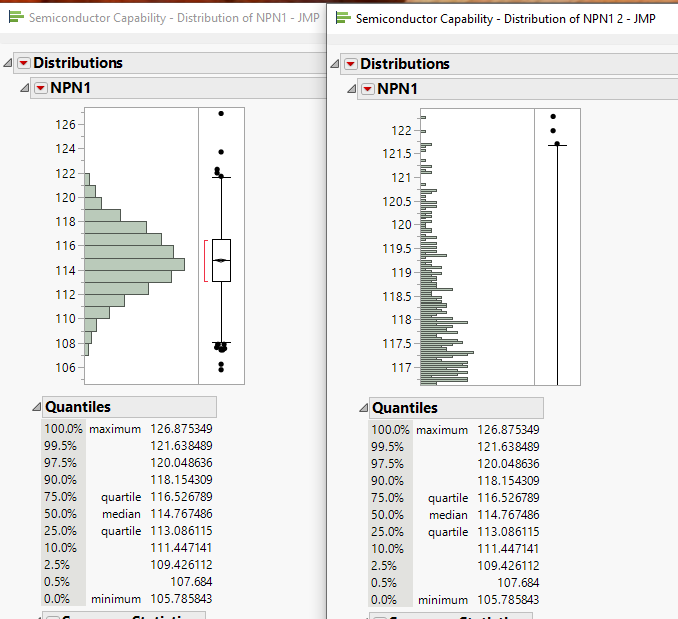Jim
Highlighted

## Re: Transforming distributions to highlight spread of values

Hi @txnelson

Thanks for the feedback. Yeah, I have looked into that, but it's not really what I'm after.

What I think I need to do is actually transform the data, but I'm not sure what the best way to do it is. If I try the "standard" options of transforming the data, the resulting distributions don't distribute out the data across a broader range.

For example, in the last set of distribution images I posted, the highlighted bin contains only 3 different integer values within that bin (65, 66, 67) [it's hard to see, I know]. But, what I'd like to do is transform it so that it has more of a continuous distribution from say, 0 to 10. If I transform the data with a log (ln) function, it still is only binning those three integers into three new non-integer rational numbers, not transforming the integer into a continuous distribution.

I think what I'd like to do is transform the integers, e.g. all values of 66, into a continuous distribution, but do this to the entire set of integers, which span the range 27 to 90. Is there a way to transform integers into a probability?

Thanks for the feedback and help!,

DS

Highlighted

## Re: Transforming distributions to highlight spread of values

I don't think there is any "best" way to do what you want to do. Since you are *ADDING* variability, you will need to make some assumptions. For example, suppose the value is 65. We have to assume that the 65 is "correct" but greater precision is not available due to measurement error (or reporting round-off or something like that). One way to accomplish this is to create a column of random normal variables with a mean of zero and a standard deviation of approximately 0.33. Now add this column of random numbers to your integer values. This should "convert" the integers into a continuous scale. I used a standard deviation of 0.33 because 3*.33 is 1, so it should be adding numbers roughly between -1 and +1. Therefore, you should still be reasonably close to the integer value.

As a proof of concept, I created a column of 100 integers, called X. I created the RanNor column as a set of random normal numbers. Finally NewX is simply X + RanNor. Here are the results: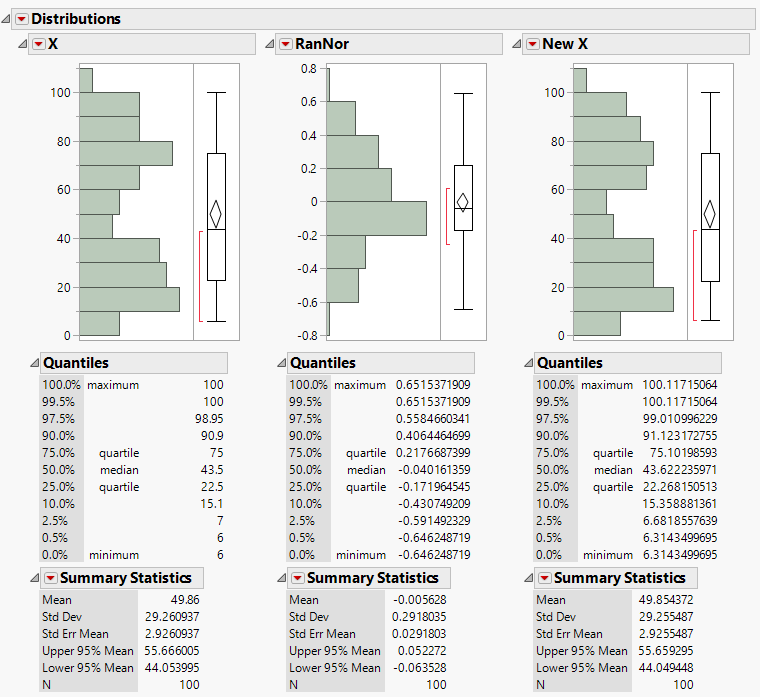NewX has a very similar shape to X, but you can see by the statistics that it does indeed have some "additional" variability without massively altering the statistics of the original data.

Is this what you are looking for??

I should add that the histograms should look similar since the purpose is to change the data, but not so much as to alter the message the data convey. To show more of the bars like you wanted in your original post, you will need to change the bin width as TXNelson suggested. Then you will see the spread around each of the integer values.

Dan Obermiller
Highlighted

## Re: Transforming distributions to highlight spread of values

It sounds like what you need to do, is to use the ability in JMP to generate your own graph, of whatever design you want it to be.  You use JSL for this.
And yes, you can get the probability of a given distribution by using
Normal Distribution() function or the xxx Distribution() function for the distribution you are interested in.  Here is an example of the Normal Distribution() function

``````Names Default To Here( 1 );
New Window( "Example: Normal Distribution",
y = Graph Box(
Y Scale( 0, 1 ),
X Scale( -4, 4 ),
XName( "q" ),
Pen Color( "red" );
Y Function( Normal Distribution( q ), q );
)
);``````
Jim
Highlighted

## Re: Transforming distributions to highlight spread of values

Hi @txnelson and @Dan_Obermiller,

Your suggestions were very helpful, and I do believe this is the direction I need to go.

I'm thinking that I might need to modify things a bit, though. I'm thinking that in order to best try and preserve the distribution of the original data, to try and do something like you suggest, but with a normal mixture function instead. As @txnelson pointed out, I am basically trying to take my CDF, which shows the integer property of my data as a stepped-CDF, and turn it into a continuous function.

I can get the location, dispersion, and probability values of a normal 3-mixture fit (from the continuous fit option in the distribution platform) and use those as the vector inputs to the normal mixture function, but I'm not sure if this is appropriate. For example, I'm not sure if I should work off the original data, a standardized version of it, or a centered version.  All version result in a normal 3 mixture as the best fit, but with different dispersions and locations (probabilities are all the same, which is not too surprising.

Ultimately, I need to take the final distribution and feed it to a logist function so I can get a probability of an event as either a yes/no and evaluate the IfMax of the yes/no logist functions (there are too many levels in my column to assign a profit matrix to the data).

Thanks for your feedback and input as it helps to ponder the best approach for analyzing my data.

DS

Article Labels

There are no labels assigned to this post.